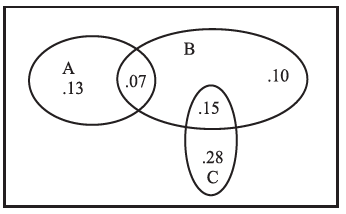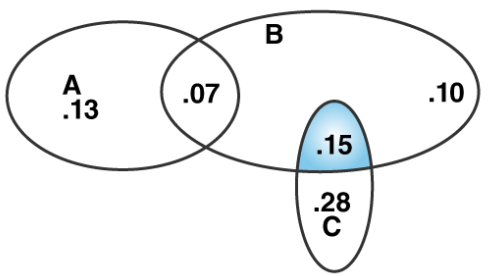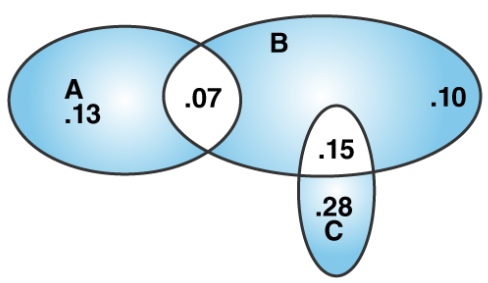The accompanying Venn diagram shows three events, A, B, and C, and also the probabilities of the various intersections (for instance, P (A ∩ B) = .07. Determine
(a) P (B ∩ C)
(b) Probability of exactly one of the three occurs.
The accompanying Venn diagram shows three events, A, B, and C, and also the probabilities of the various intersections (for instance, P (A ∩ B) = .07. Determine
(a) P (B ∩ C)
(b) Probability of exactly one of the three occurs.Solution:

It is given thatFrom the Venn Diagram given

(a)From the Venn diagram we have(b) Probability of exactly one of the three occursP (exactly one of the three occurs)